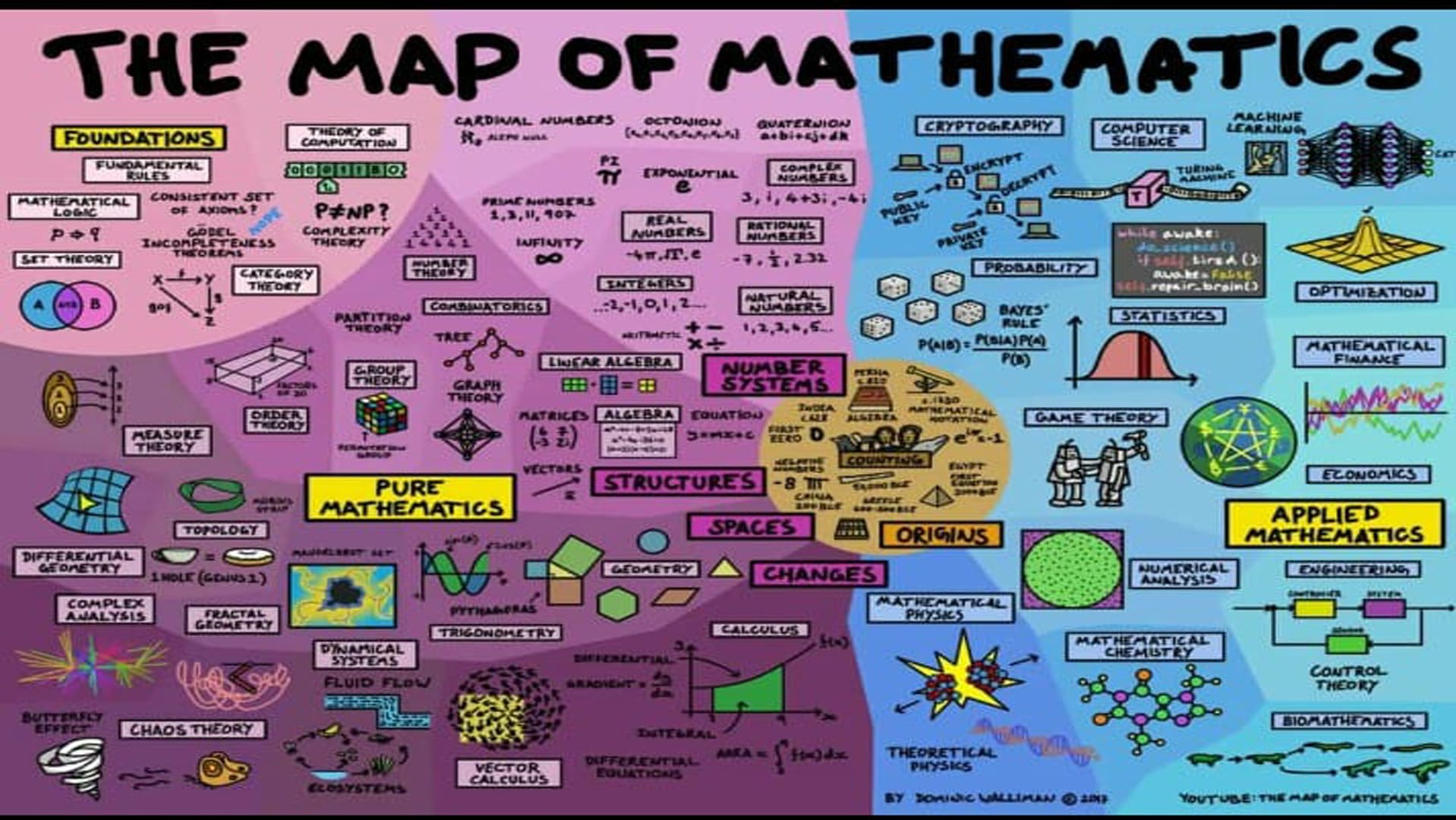Other

Mathematics Teacher

I am offering to help you in your Mathematical work with full Quality and Efficiency. I will help you to understand the basic concept behind the problems and I will make it easy for you to solve them. As a experienced math tutor I have a good grip in all the subjects related to math, I will Guide you through your math problems and will explain each solution step-by-step.I can provide you notes in PDF form and handwritten scanned form , I can help you with Calculus Trigonometry Geometry Algebra Linear Algebra Discrete Math Complex Number Systems of Equations General Math Differential Equation Derivation Integration Set Theory Number Theory\$10/hr Ongoing